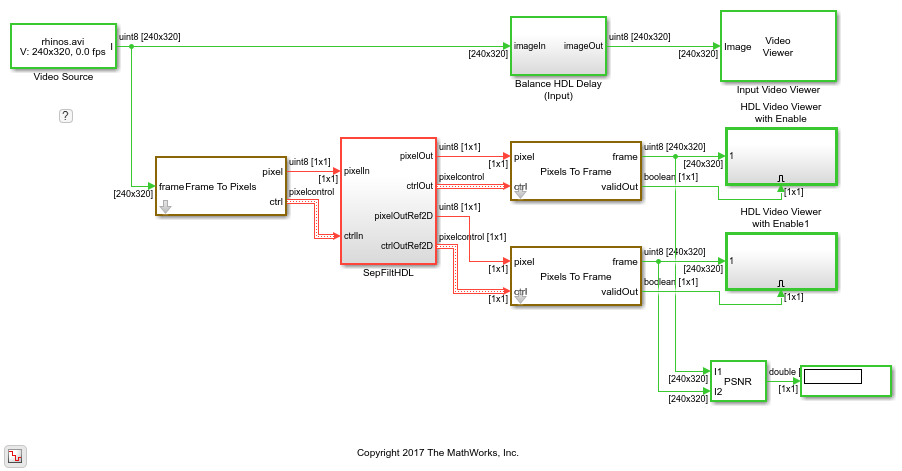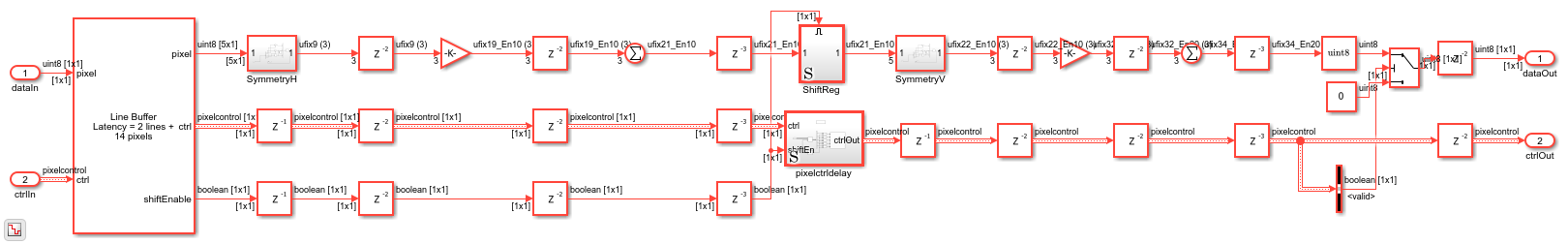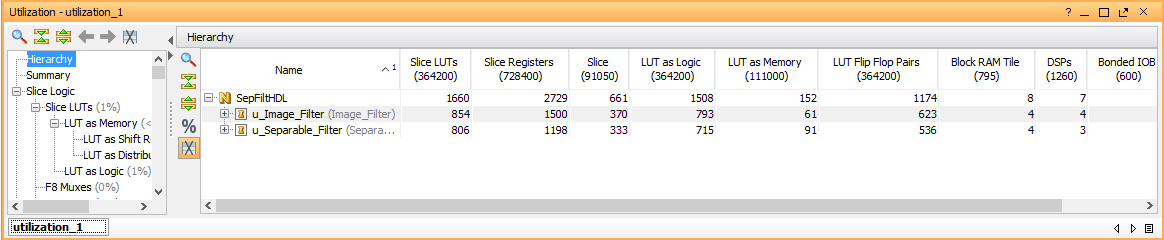# Using the Line Buffer to Create Efficient Separable Filters

This example shows how to design and implement a separable image filter, which uses fewer hardware resources than a traditional 2-D image filter.

Traditional image filters operate on a region of pixels and compute the resulting value of one central pixel using a two-dimensional filter kernel which is a matrix that represents the coefficients of the filter. Each coefficient is multiplied with its corresponding pixel and the result is summed to form the value. The region is then moved by one pixel and the next value is computed.

A separable filter is simple in concept: if the two-dimensional filter kernel can be factored into a horizontal component and a vertical component, then each direction can be computed separately using one-dimensional filters. This factorization can only be done for certain types of filter kernels. These kernels are called separable since the parts can be separated. Deciding which kernels are separable and which are not is easy using linear algebra in MATLAB. Mathematically, the two 1-D filters convolve to equal the original 2-D filter kernel, but a separable filter implementation often saves hardware resources.

### Introduction

The SeparableFilterHDL.slx system is shown below. The SeptFiltHDL subsystem contains the separable filter, and also an Image Filter block implementation of the equivalent 2-D kernel as a reference.

```modelname = 'SeparableFilterHDL'; open_system(modelname); set_param(modelname,'SampleTimeColors','on'); set_param(modelname,'SimulationCommand','Update'); set_param(modelname,'Open','on'); set(allchild(0),'Visible','off'); ```### Determine Separable Filter Coefficients

Start by deciding what the purpose of your filter will be and compute the kernel. This example uses a Gaussian filter of size 5x5 with a standard deviation of 0.75. This filter has a blurring effect on images and is often used to remove noise and small details before other filtering operations, such as edge detection. Notice that the Gaussian filter kernel is circularly symmetric about the center.

```Hg = fspecial('gaussian',[5,5],0.75) ```
```Hg = 0.0002 0.0033 0.0081 0.0033 0.0002 0.0033 0.0479 0.1164 0.0479 0.0033 0.0081 0.1164 0.2831 0.1164 0.0081 0.0033 0.0479 0.1164 0.0479 0.0033 0.0002 0.0033 0.0081 0.0033 0.0002 ```

To check if the kernel is separable, compute its rank, which is an estimate of the number of linearly independent rows or columns in the kernel. If `rank` returns 1, then the rows and columns are related linearly and the kernel can be separated into its horizontal and vertical components.

```rankHg = rank(Hg) ```
```rankHg = 1 ```

To separate the kernel, use the `svd` function to perform singular value decomposition. The `svd` function returns three matrices, `[U,S,V]`, such that `U*S*V'` returns the original kernel, `Hg`. Since the kernel is rank 1, `S` contains only one non-zero element. The components of the separated filter are the first column of each of `U` and `V`, and the singular value split between the two vectors. To split the singular value, multiply both vectors with the square root of `S`. You must reshape `V` so that `Hh` is a horizontal, or row, vector.

For more information on filter separability, refer to the links at the bottom of this example.

```[U,S,V]=svd(Hg) Hv=abs(U(:,1)*sqrt(S(1,1))) Hh=abs(V(:,1)'*sqrt(S(1,1))) ```
```U = -0.0247 -0.9912 -0.1110 0.0673 -0.0000 -0.3552 0.0737 -0.6054 -0.0431 0.7071 -0.8640 -0.0301 0.4988 0.0619 -0.0000 -0.3552 0.0737 -0.6054 -0.0431 -0.7071 -0.0247 -0.0754 0.0761 -0.9939 0.0000 S = 0.3793 0 0 0 0 0 0.0000 0 0 0 0 0 0.0000 0 0 0 0 0 0.0000 0 0 0 0 0 0.0000 V = -0.0247 0.1742 0.8521 -0.4929 0 -0.3552 0.5980 -0.0716 0.1053 0.7071 -0.8640 -0.4937 0.0199 -0.0968 0.0000 -0.3552 0.5980 -0.0716 0.1053 -0.7071 -0.0247 -0.1031 0.5131 0.8518 -0.0000 Hv = 0.0152 0.2188 0.5321 0.2188 0.0152 Hh = 0.0152 0.2188 0.5321 0.2188 0.0152 ```

You can check your work by reconstructing the original kernel from these factored parts and see if they are the same to within floating-point precision. Compute the check kernel, `Hc`, and compare it to the original `Hg` using a tolerance.

```Hc = Hv * Hh; equalTest = all(Hc(:)-Hg(:) < 5*max(eps(Hg(:)))) ```
```equalTest = logical 1 ```

This result proves that `Hv` and `Hh` can be used to recreate the original filter kernel.

### Fixed-Point Settings

For HDL code generation, you must set the filter coefficients to fixed-point data types. When picking fixed-point types, you must consider what happens to separability when you quantize the kernel.

First, quantize the entire kernel to a nominal data type. This example uses a 10-bit fixed-point number. Let the fixed-point tools select the best fraction length to represent the kernel values. Do the same conversion for the horizontal and vertical component vectors.

```Hgfi = fi(Hg,0,10); Hvfi = fi(Hv,0,10); Hhfi = fi(Hh,0,10); ```

In this case, the best-precision 10-bit answer for `Hg` has 11 fractional bits, while `Hv` and `Hh` use only 10 fractional bits. This result makes sense since `Hv` and `Hh` are multiplied and added together to make the original kernel.

Now you must check if the quantized kernel is still rank 1. Since the `rank` and `svd` functions do not accept fixed-point types, you must convert back to doubles. This operation does not quantize the results, as long as the fixed-point type is smaller than 53 bits, which is the effective mantissa size of doubles.

```rankDouble = rank(double(Hgfi)) ```
```rankDouble = 3 ```

This result shows that quantization can have a dramatic effect on the separability: since the rank is no longer 1, the quantized filter does not seem to be separable. For this particular filter kernel, you could experiment with the quantized word-length and discover that 51 bits of precision are needed in order for the rank function to return 1 after quantization. Actually, this result is overly conservative because of quantization of near-zero values within the `rank` function.

Instead of expanding the fixed-point type to 51 bits, add a tolerance argument to the `rank` function to limit the quantization effects.

```rankDouble2048 = rank(double(Hgfi),1/2048) ```
```rankDouble2048 = 1 ```

This result shows that the quantized kernel is rank 1 within an 11-bit fractional tolerance. So, the 11-bit separated coefficients are acceptable after all.

Another quantization concern is whether the fixed-point filter maintains flat field brightness. The filter coefficients must sum to 1.0 to maintain brightness levels. For a normalized Gaussian filter such as this example, the coefficient sum is always 1.0, but this sum can be moved away from 1.0 by fixed-point quantization. It can be critical in video and image processing to maintain exactly 1.0, depending on the application. If you imagine a chain of filters, each one of which raises the average level by around 1%, then the cumulative error can be large.

```sumHg = sum( Hg(:) ) sumHgfi = sum( Hgfi(:) ) ```
```sumHg = 1.0000 sumHgfi = 1 DataTypeMode: Fixed-point: binary point scaling Signedness: Unsigned WordLength: 15 FractionLength: 11 ```

In this case, the sums of the double-precision `Hg` and the fixed-point `Hgfi` are indeed 1.0. If maintaining brightness levels to absolute limits is important in your application, then you might have to manually adjust the filter coefficient values to maintain a sum of 1.

Finally, check that the combination of the quantized component filters still compares to the quantized kernel. By default, the `fi` function uses full precision on the arithmetic expression. Use convergent rounding since there are some coefficient values very near the rounding limit.

```Hcfi = fi(Hvfi * Hhfi,0,10,'fimath',fimath('RoundingMethod','Convergent')); equalTest = all( Hcfi(:)==Hgfi(:) ) ```
```equalTest = logical 1 ```

This result confirms that the fixed-point, separated coefficients achieve the same filter as the 2-D Gaussian kernel.

### Implementing the Separable Filter

To see the separable filter implementation, open the Separable Filter subsystem that is inside the SepFiltHDL subsystem.

```open_system([modelname '/SepFiltHDL/Separable Filter'],'force'); ```This subsystem selects vertical and horizontal vectors of pixels for filtering, and performs the filter operation.

The Line Buffer outputs a column of pixels for every time step of the filter. The Line Buffer also pads the edges of the image. This model uses Padding method: `Constant`, with a value of 0. The shiftEnable output signal is normally used to control a horizontal shift register to compile a 2-D pixel kernel. However, for a separable filter, you want to work in each direction separately. This model uses the output pixel column for the vertical filter, and uses the shiftEnable signal later to construct the horizontal pixel vector.

The separated horizontal and vertical filters are symmetric, so the model uses a pre-adder to reduce the number of multipliers even further. After the adder, a Gain block multiplies the column of pixels by the `Hv` vector. The Gain parameter is set to `Hv` and the parameter data type is `fixdt(0,10)`. The resulting output type in full-precision is `ufix18_En10`. Then a Sum block completes the vertical filter. The Sum block is configured in full-precision mode. The output is a scalar of `ufix21_En10` type.

There are many pipelining options you could choose, but since this design is simple, manual pipelining is quick and easy. Adding delays of 2 cycles before and after the Gain multipliers ensures good speed when synthesized for an FPGA. A delay of 3 cycles after the Sum allows for it to be sufficiently pipelined as well. The model balances these delays on the pixelcontrol bus and the shiftEnable signal before going to the horizontal dimension filter.

The best way to create a kernel-width shift register is to use a Tapped Delay block, which shifts in a scalar and outputs the register values as a vector. For good HDL synthesis results, use the Tapped Delay block inside an enabled subsystem, with the Synchronous marker block.

The output of the Tapped Delay subsystem is a vector of 5 horizontal pixels ready for the horizontal component of the separable filter. The model implements a similar symmetric pre-add and Gain block, this time with `Hh` as the parameter. Then, a Sum block and similar pipelining complete the horizontal filter. The final filtered pixel value is in the full-precision data type `ufix34_En20`.

Many times in image processing you would like to do full-precision or at least high-precision arithmetic operations, but then return to the original pixel input data type for the output. This subsystem returns to the original pixel type by using a Data Type Conversion block set to `uint8`, with `Nearest` rounding and saturation.

The Vision HDL Toolbox blocks force the output data to zero when the output is not valid, as indicated in the pixelcontrol bus output. While not strictly required, this behavior makes testing and debugging much easier. To accomplish this behavior, the model uses a Switch block with a Constant block set to 0.

### Resource Comparison

The separable 5x5 filter implementation uses 3 multipliers in the vertical direction and 3 multipliers in the horizontal direction, for a total of 6 multipliers. A traditional image filter usually requires 25 multipliers for a 5x5 kernel. However, the Image Filter block takes advantage of any symmetry in the kernel. In this example the kernel has 8-way and 4-way symmetry, so the Image Filter only uses 5 multipliers. In general there are savings in multipliers when implementing a separable filter, but in this case the 2-D implementation is similar.

The separable filter uses 4 two-input adders in each direction, 2 for the pre-add plus 2 in the Sum, for a total of 8. The Image Filter requires 14 adders total, with 10 pre-add adders and 4 final adders. So there is a substantial saving in adders.

The Image Filter requires 25 registers for the shift register, while the separable filter uses only 5 registers for the shift register. Each adder also requires a pipeline register so that is 8 for the separable case and 14 for the traditional case. The number of multiplier pipeline registers scales depending on the number of multipliers.

The separable filter uses fewer adders and registers than the 2-D filter. The number of multipliers is similar between the two filters only because the 2-D implementation optimizes the symmetric coefficients.

### Results of the Simulation

The resulting images from the simulation of the separable filter and the reference Image Filter are very similar. Using the fixed-point settings in this example, the difference between the separable filter and the reference filter never exceeds one bit. This difference is a 0.1% difference or greater than 54 dB PSNR between the filtered images overall.

### HDL Code Generation

To check and generate the HDL code referenced in this example, you must have an HDL Coder™ license.

To generate the HDL code, use the following command.

```makehdl('SeparableFilterHDL/SepFiltHDL') ```

To generate the test bench, use the following command. Note that test bench generation takes a long time due to the large data size. Reduce the simulation time before generating the test bench.

```makehdltb('SeparableFilterHDL/SepFiltHDL') ```

The part of this model that you can implement on an FPGA is the part between the Frame To Pixels and Pixels To Frame blocks. The SepFiltHDL subsystem includes both the separable algorithm and the traditional 2-D implementation for comparison purposes.

### Simulation in an HDL Simulator

Now that you have HDL code, you can simulate it in your HDL simulator. The automatically generated test bench allows you to prove that the Simulink simulation and the HDL simulation match.

### Synthesis for an FPGA

You can also synthesize the generated HDL code in an FPGA synthesis tool, such as Xilinx Vivado. In a Virtex-7 FPGA (xc7v585tffg1157-1), the filter design achieves a clock rate of over 250 MHz.

The utilization report shows that the separable filter uses fewer resources than the traditional image filter. The difference in resource use is small due to the symmetry optimizations applied by the Image Filter block.### Going Further

The filter in this example is configured for Gaussian filtering but other types of filters are also separable, including some that are very useful. The mean filter, which has a kernel with coefficients that are all `1/N`, is always separable.

```Hm = ones(3)./9 rank(Hm) ```
```Hm = 0.1111 0.1111 0.1111 0.1111 0.1111 0.1111 0.1111 0.1111 0.1111 ans = 1 ```

Or the Sobel edge-detection kernel:

```Hs = [1 0 -1; 2 0 -2; 1 0 -1] rank(Hs) ```
```Hs = 1 0 -1 2 0 -2 1 0 -1 ans = 1 ```

Or gradient kernels like this:

```Hgrad = [1 2 3; 2 4 6; 3 6 9] rank(Hgrad) ```
```Hgrad = 1 2 3 2 4 6 3 6 9 ans = 1 ```

Separability can also be applied to filters that do not use multiply-add, such as morphological filters where the operator is min or max.

### Conclusion

You have used linear algebra to determine if a filter kernel is separable or not, and if it is, you learned how to separate the components using the `svd` function.

You explored the effects of fixed-point quantization and learned that it is important to work with precise values when calculating rank and singular values. You also learned about the importance of maintaining DC gain. Finally you learned why separable filters can be implemented more efficiently and how to calculate the savings.

### References

 Eddins, S. "Separable convolution". Steve on Image Processing (October 4, 2006).

 Eddins, S. "Separable convolution: Part 2". Steve on Image Processing (November 28, 2006).